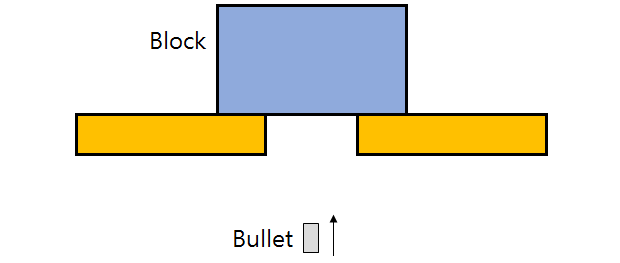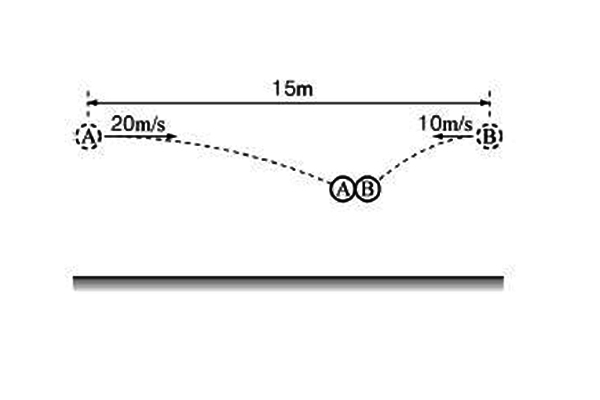Classical Mechanics

# Inelastic collisions

A pickup truck with mass $2.0 \times 10^3\text{ kg}$ is traveling eastbound at $+24\text{ m/s},$ while a compact car with mass $1.0 \times 10^3\text{ kg}$ is traveling westbound at $-24\text{ m/s}$ on the same road. The truck and car collide and thereafter move as a single, tangled mass. Find the change in the kinetic energy of the system consisting of both vehicles.A $20\text{ g}$ bullet shot vertically upward with constant speed $2000\text{ m/s}$ passes through the center of gravity of a $5.0\text{ kg}$ block at rest, as shown in the above diagram. The bullet moves at $400\text{ m/s}$ right after it passes through the block. How high would the block move from its initial position?

Take the acceleration of gravity as $10\text{ m/s}^2.$
Assume that the effect on the bullet by gravity is negligible.

A bullet with mass $13.0\text{ g}$ and speed $434\text{ m/s}$ hits a wooden block of mass $390.0\text{ g}$ on a frictionless table. This bullet comes to a complete stop inside the wooden block. What is the approximate speed of the wooden block and bullet after the bullet stops inside the block?

Assume that the mass loss of the block by the bullet is negligible.

A bullet with mass $4.40\text{ g}$ and speed $773\text{ m/s}$ flying horizontally hits a wooden block of mass of $520\text{ g}$ on a frictionless table. Now the bullet passes through the wooden block with a reduced speed of $513\text{ m/s}.$ What is the speed of the wooden block after the bullet completely passes through it?

Assume that mass loss of the block from the bullet is negligible.In the above figure, two objects $A$ and $B$ with the same mass $m$ are $15$ m away from each other. Now, $A$ and $B$ are thrown horizontally at the same time at the velocities $20$ m/s and $-10$ m/s, respectively, eventually colliding with each other in the air. If the two objects stick together after a perfectly inelastic collision, what is the speed of the mass at the moment of collision (in m/s)?

Assume that gravitational acceleration is $g= 10$ m/s$^{2}$.

×